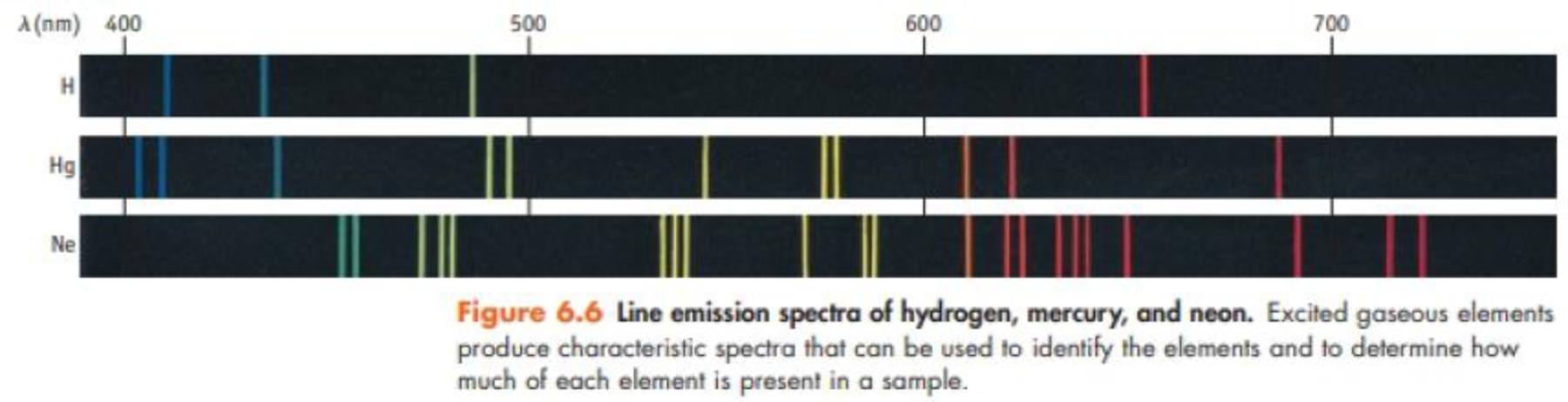# The most prominent line in the spectrum of mercury is at 253.652 nm. Other lines are located at 365.015 nm, 404.656 nm, 435.833 nm, and 101.975 nm. (a) which of these lines represents the most energetic light? (b) what is the frequency of the most prominent line? What is the energy of one photon with this wavelength? (c) Are any of these lines found in the spectrum of mercury shown in figure 6.6? What color or colors are these lines?### Chemistry & Chemical Reactivity

9th Edition
John C. Kotz + 3 others
Publisher: Cengage Learning
ISBN: 9781133949640

#### Solutions

Chapter
Section### Chemistry & Chemical Reactivity

9th Edition
John C. Kotz + 3 others
Publisher: Cengage Learning
ISBN: 9781133949640
Chapter 6, Problem 13PS
Textbook Problem
50 views

## The most prominent line in the spectrum of mercury is at 253.652 nm. Other lines are located at 365.015 nm, 404.656 nm, 435.833 nm, and 101.975 nm.(a) which of these lines represents the most energetic light?(b) what is the frequency of the most prominent line? What is the energy of one photon with this wavelength?(c) Are any of these lines found in the spectrum of mercury shown in figure 6.6? What color or colors are these lines?(a)

Interpretation Introduction

Interpretation: The most energetic line among the given lines in the emission spectrum of mercury has to be identified.

Concept introduction:

• Planck’s equation,

E==hcλwhere, E=energyh=Planck'sconstantν=frequency

The energy increases as the wavelength of the light decrease. Also the energy increases as the frequency of the light increases.

• The frequency of the light is inversely proportional to its wavelength.

ν=cλwhere, c=speedoflightν=frequencyλ=wavelength

### Explanation of Solution

Emission spectrum of mercury has five lines in 253.652nm, 365.015nm, 404.656nm, 435.833nm and 1013.975nm where 253.652nm is prominent

(b)

Interpretation Introduction

Interpretation: The frequency and the energy per photon of most energetic line in the emission spectrum of mercury have to be calculated.

Concept introduction:

• Planck’s equation,

E==hcλwhere, E=energyh=Planck'sconstantν=frequency

The energy increases as the wavelength of the light decrease. Also the energy increases as the frequency of the light increases.

• The frequency of the light is inversely proportional to its wavelength.

ν=cλwhere, c=speedoflightν=frequencyλ=wavelength

(c)

Interpretation Introduction

Interpretation: Any of the given line is whether found in the spectrum of mercury has to be identified and listed.

Concept introduction:

• Electromagnetic radiations are a type of energy surrounding us. They are of different types like radio waves, IR, UV, X-ray etc.
• Visible light lies in the wavelength of 400nm to 700nm.

### Still sussing out bartleby?

Check out a sample textbook solution.

See a sample solution

#### The Solution to Your Study Problems

Bartleby provides explanations to thousands of textbook problems written by our experts, many with advanced degrees!

Get Started